Euler-Poisson-Darboux equation

The second-order hyperbolic partial differential equation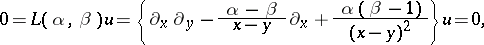whereandare real positive parameters such that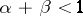(see [a8]) and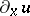denotes the partial derivative of the functionwith respect to.

This equation appears in various areas of mathematics and physics, such as the theory of surfaces [a4], the propagation of sound [a3], the colliding of gravitational waves [a6], etc.. The Euler–Poisson–Darboux equation has rather interesting properties, e.g. in relation to Miller symmetry and the Laplace sequence, and has a relation to, e.g., the Toda molecule equation (see [a4]).

A formal solution to the Euler–Poisson–Darboux equation has the form [a8]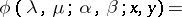where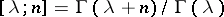and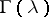is the gamma-function.

By conjugate transformation of the differential operator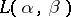with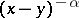one obtains the operator(a1)

Many papers deal with the equation(a2)

(see, e.g., [a11], [a8], [a7], [a10], [a12]). In the characteristic triangleand under the conditions(a3)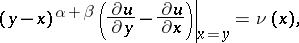the solution of (a2) can be expressed as (see [a12]):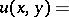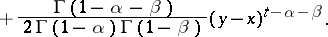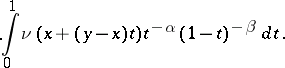Formulas for the general solution of (a2) are known for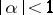,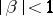;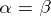; and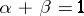. For other values of the parameters, an explicit representation of the solution can be given using a regularization method for the divergent integral (see [a7]). The unique solvability of a boundary value problem for (a2) with a non-local boundary condition, containing the Szegö fractional integration and differentiation (cf. Fractional integration and differentiation) operators, is proved in [a11]. For (a2) local solutions, propagation of singularities, and holonomic solutions of hypergeometric type are studied in [a14]. For hypergeometric functions of several variables occurring as solutions of boundary value problems for (a2), see also [a14].

A-difference analogue of the operator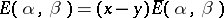is considered in [a8]; it has been proved that the-deformation of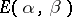is the-difference operator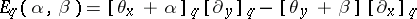.

The existence and uniqueness of global generalized solutions of mixed problems for the generalized Euler–Poisson–Darboux equation(a4)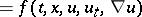are studied in [a15], using Galerkin approximation. Moreover, the classical solution of (a4) has been obtained by using properties of Sobolev spaces and imbedding theorems (cf. also Imbedding theorems). See [a2], [a11], [a1], [a9] for various aspects of (a4).

See [a5] for necessary and sufficient conditions for stabilization of the solution of the Cauchy problem for the Euler–Poisson–Darboux equation in a homogeneous symmetric space.